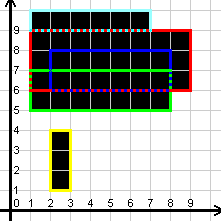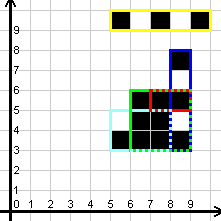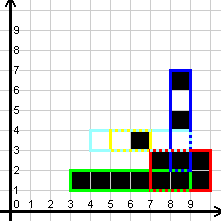Problem Statement

NOTE: This problem statement contains images that may not display properly if viewed outside of the applet.

There is an infinite sheet of paper which is divided into 1x1 cells. A cartesian coordinate system exists on the sheet, with its origin at the corner of one of the cells. The X-axis goes from left to right in the positive direction and the Y-axis goes from bottom to top in the positive direction. The axes are parallel to the sides of the cells.

Initially, all the cells are white. You are given a String[] rectangles, each element of which represents a rectangular pattern you will draw on the sheet. Each rectangle is formatted "x1 y1 x2 y2 type" (quotes for clarity), where (x1, y1) is the bottom left corner of the rectangle, (x2, y2) is the top right corner of the rectangle, and type is the number of one of the following patterns:

• Pattern 1: All cells are colored black.
• Pattern 2: All cells in odd-numbered rows are colored black (the rows of the rectangle are numbered from bottom to top, starting from 0).
• Pattern 3: All cells in odd-numbered columns are colored black (the columns of the rectangle are numbered from left to right, starting from 0).
• Pattern 4: All cells with row and column numbers that are either both odd or both even are colored black.

The pictures below illustrate a rectangle and all the possible patterns:Return the total number of black cells on the sheet of paper after all the rectangles are drawn.

Definition

 Class: BlackWhiteRectangles Method: blackCount Parameters: String[] Returns: int Method signature: int blackCount(String[] rectangles) (be sure your method is public)

Constraints

-rectangles will contain between 1 and 50 elements, inclusive.
-Each element of rectangles will be formatted as "x1 y1 x2 y2 type" (quotes for clarity), where x1, y1, x2, y2 will each be an integer between 1 and 40000, inclusive, without leading zeros, and type will be an integer between 1 and 4, inclusive, without leading zeros.
-In each element of rectangles, x1 will be strictly less than x2, and y1 will be strictly less than y2.

Examples

0)

 {"1 1 6 8 1", "11 1 16 8 2", "1 11 6 18 3", "11 11 16 18 4"}
Returns: 82
 Here we have 4 non-overlapping 5x7 rectangles with all possible filling patterns. Therefore the total number of black cells is 35 + 14 + 15 + 18 = 82.
1)

 {"1 1 11 11 1", "1 1 11 11 2", "9 9 19 19 3", "9 9 19 19 4"}
Returns: 172
 In this case we have 2 equal 10x10 rectangles with filling patterns 1 and 2 (100 black cells) and 2 equal 10x10 rectangles with filling patterns 3 and 4 (75 black cells). Since the first two rectangles and the last two rectangles have 3 black cells in common, the result is 100 + 75 - 3 = 172.
2)

 {"1 6 9 9 1", "1 9 7 10 1", "1 5 8 7 1", "2 6 8 8 1", "2 1 3 4 1"}
Returns: 403)

 {"7 5 9 6 4", "6 3 9 6 4", "8 3 9 8 4", "5 9 10 10 4", "5 3 8 5 4"}
Returns: 134)

 {"7 1 10 3 1", "8 2 9 7 4", "5 3 7 4 3", "4 3 9 4 2", "3 1 9 2 1"}
Returns: 13Problem url:

http://www.topcoder.com/stat?c=problem_statement&pm=8142

Problem stats url:

http://www.topcoder.com/tc?module=ProblemDetail&rd=12008&pm=8142

ivan_metelsky

Testers:

PabloGilberto , radeye , vorthys , Olexiy

Problem categories:

Geometry, Simple Math